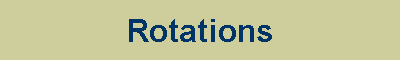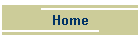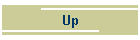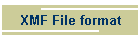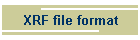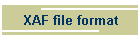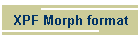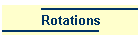# Rotations in IMVU or Cal3D

## A few simple rotations:

### No rotation at all

 0 0 0 -1 0 0 0 1

### Rotation about the X axis

 Angle Values Alternative Values 90° -0.707 0 0 -0.707 0.707 0 0 0.707 180° -1 0 0 0 1 0 0 0 270° 0.707 0 0 -0.707 -0.707 0 0 0.707

### Rotation about the Y axis

 Angle Values 90° 0 -0.707  0 -0.707 180° 0 -1  0 0 270° 0 0.707  0 -0.707

### Rotation about the Z axis

 Angle Values 90° 0 0 -0.707 -0.707 180° 0 0 -1 0 270° 0 0 0.707 -0.707

## Combining rotations

This is the difficult one: rotations are represented using a specialised form of complex number known as a quaternion. My best guess is that the number parts are meant to be read from right to left.

If we call two rotations P (P3,P2,P1,P0) and Q (Q3,Q2,Q1,Q0) and the combination R (R3,R2,R1,R0) then to determine R we need

R=P*Q

R0=P0*Q0-P1*Q1-P2*Q2-P3*Q3

For R1..3

R1=P0*Q1+P1*Q0+P2*Q3-P3*Q2

R2=P0*Q2+P2*Q0+P3*Q1-P1*Q3

R3=P0*Q3+P3*Q0+P1*Q2-P2*Q1

### Reversing or undoing a rotation

You can reverse the effect of a rotation by negating Q0 or negating Q1,Q2,Q3, either one works. Multiplying the rotation by its reverse or "conjugate" yields "0 0 0 1" or "0 0 0 -1". Note this is not the same as rotating the same joint in the other direction.

### Normalising or cleaning up

The values should be "normalised", that is to say they should satisfy  Q02+Q12+Q22+Q32=1

If you start with normalised values you should finish with normalised values, but if you perform a lot of operations they may "drift" due to rounding.